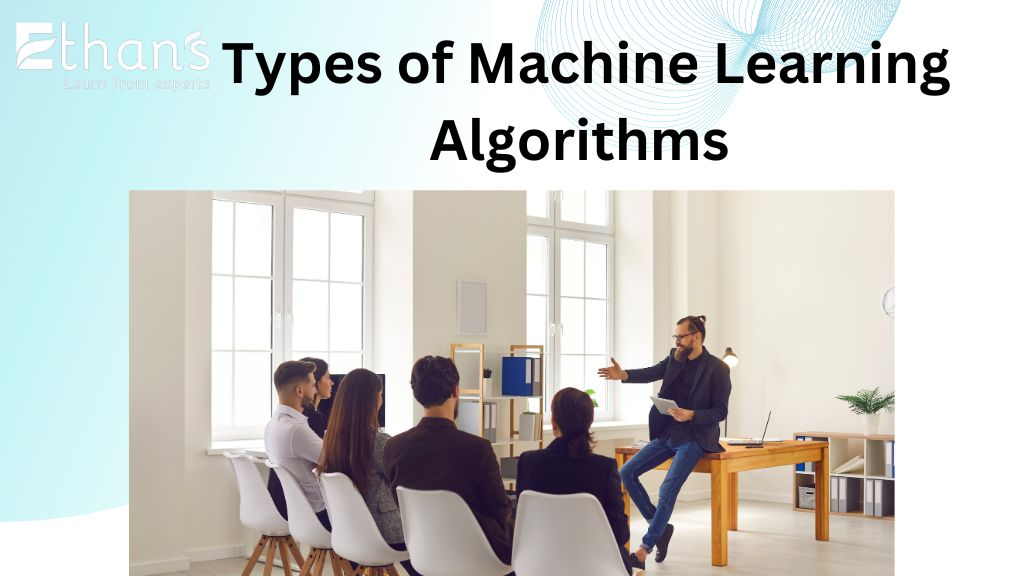Featured Categories# Learn About the Types of Machine Learning Algorithms

Machine learning algorithms are at the core of modern data analysis and decision-making processes. They enable computers to learn patterns and make predictions or decisions without being explicitly programmed. There are various machine learning algorithms, each designed to solve specific problems and utilize different techniques. In this article, we will explore the most common types of machine learning algorithms and their applications.

## Introduction

Machine learning algorithms can be broadly classified into three categories: supervised learning, unsupervised learning, and reinforcement learning. Let’s delve into each of these categories and understand the algorithms within them.

### Supervised Learning Algorithms

Supervised learning algorithms learn from labeled training data, where the input data is paired with corresponding output labels. The goal is to learn a function that maps the input to the output accurately. Here are some popular supervised learning algorithms:

Linear Regression

Linear regression is a simple yet powerful algorithm used for predicting continuous numerical values. It models the relationship between the input variables and the target variable using a linear equation. It finds the best-fit line that minimizes the sum of squared errors.

Logistic Regression

Logistic regression is a binary classification algorithm used to predict categorical outcomes. It calculates the probability of an event occurring using a logistic function. The algorithm learns the relationship between the input variables and the probability of a specific outcome.

Decision Trees

Decision trees are versatile algorithms that can be used for both classification and regression tasks. They create a tree-like model of decisions and their possible consequences. Each internal node represents a test on an attribute, each branch represents the outcome of the test, and each leaf node represents a class label or a predicted value.

Random Forests

Random forests combine multiple decision trees to make more accurate predictions. They create an ensemble of decision trees and aggregate their predictions. Random forests are known for their robustness, scalability, and ability to handle large datasets with high dimensionality.

Support Vector Machines (SVM)

Support Vector Machines are powerful algorithms used for both classification and regression tasks. They construct a hyperplane or a set of hyperplanes in a high-dimensional space that maximally separates the data points of different classes. SVMs are effective in handling complex datasets with clear margin separation.

Naive Bayes

Naive Bayes is a probabilistic algorithm based on Bayes’ theorem with strong independence assumptions between the features. Despite its simplicity, it performs remarkably well in many real-world applications. Naive Bayes is commonly used for text classification and spam filtering.

### Unsupervised Learning Algorithms

Unsupervised learning algorithms learn patterns and relationships from unlabeled data. They explore the inherent structure in the data without any predefined output labels. Let’s explore some common unsupervised learning algorithms:

K-means Clustering

K-means clustering is a popular algorithm for partitioning a dataset into K distinct non-overlapping clusters. It aims to minimize the sum of squared distances between the data points and their respective cluster centroids. K-means clustering finds application in customer segmentation, image compression, and anomaly detection.

Hierarchical Clustering

Hierarchical clustering builds a hierarchy of clusters by recursively merging or splitting them based on a similarity measure. It does not require the number of clusters to be predefined. Hierarchical clustering is useful in gene expression analysis, document clustering, and social network analysis.

Principal Component Analysis (PCA)

Principal Component Analysis is a dimensionality reduction technique used to identify patterns and capture the most important features in high-dimensional data. It transforms the original variables into a new set of uncorrelated variables called principal components. PCA is widely used in image recognition, data visualization, and feature extraction.

Association Rule Learning

Association rule learning aims to discover interesting relationships or associations among items in large datasets. It identifies frequent itemsets and generates rules that represent relationships between items. Association rule learning is commonly used in market basket analysis, recommendation systems, and web mining.

### Reinforcement Learning Algorithms

Reinforcement learning algorithms learn through interaction with an environment to maximize a reward signal. They focus on making sequential decisions and learn from feedback in the form of rewards or punishments. Let’s explore some popular reinforcement learning algorithms:

Q-Learning

Q-Learning is a model-free reinforcement learning algorithm that learns by updating the action-value function based on the observed rewards. It uses a table or a function approximation to store the expected cumulative rewards for different state-action pairs. Q-Learning is widely used in robotics, game-playing agents, and autonomous systems.

Deep Q-Networks (DQN)

Deep Q-Networks combine Q-Learning with deep neural networks to handle high-dimensional state spaces. They approximate the action-value function using a deep neural network and update the network parameters through gradient descent. Deep Q-Networks have achieved remarkable results in playing video games and controlling complex systems.

Policy gradient methods aim to learn a parameterized policy directly by optimizing a performance measure. They use gradient ascent to update the policy parameters based on the observed rewards. Policy gradient methods have been successful in training autonomous agents, optimizing robotic control, and natural language processing.

## Conclusion

Machine learning algorithms come in various types, each serving different purposes in solving data-driven problems. Supervised learning algorithms are useful for prediction and classification tasks, while unsupervised learning algorithms are effective in pattern recognition and clustering. Reinforcement learning algorithms excel in sequential decision-making problems. Understanding these types of algorithms is crucial for choosing the right approach for different applications.

### Latest Post

#### Presenting AWS’s Latest Credential: Data Engineer Associate Certification

September 29, 2023

#### 10 Short-Term Job-Oriented Courses to Kickstart Your Career

September 28, 2023

#### Exploring Python Inheritance: Understanding Various Types of Inheritance in Python

September 22, 2023

#### Top 30 Python Interview Questions for Aspiring Developers

September 15, 2023

August 24, 2023

August 23, 2023

August 22, 2023

August 8, 2023

August 4, 2023

August 3, 2023

August 2, 2023

July 31, 2023